# Gnuplotting

Create scientific plots using gnuplot

December 1st, 2012 | 7 Comments

In an earlier entry we created a vector field from measured data. Now we will visualize functions with the `vector` plotting style. As functions we have two 1/r potentials which define the amplitude of the vectors, as can be seen in Fig. 1. It is often better to indicate the amplitude by a color instead of by the length of the single vectors, especially if there are big changes. For the exact functions have a look into the corresponding file.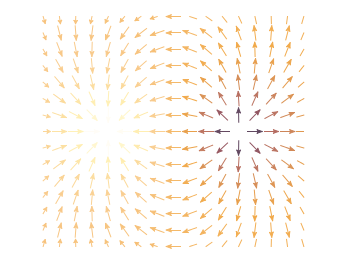Fig. 1 Vector field of two sources with the opposite charge. The color indicates the amplitude. (code to produce this figure)

By analogy to the data vector field we have again a dx, and dy function for the length of the vectors.

```dx(x,y) = scaling*ex(x,y)/enorm(x,y)
dy(x,y) = scaling*ey(x,y)/enorm(x,y)
```

Now we need a trick, because we have to fill the `u 1:2:3:4` for the vector style with our function data. The four columns are then x,y,dx,dy of the vectors, where dx, dy are the lengths of the vector in x and y direction. Here the special filename `++` is a big help. It gives us x and y points as a first and second column, which we could address by `\$1` and `\$2`. The number of points for the two axes are handled by the `samples` and `isosamples` command.

```set samples 17    # x-axis
set isosamples 15 # y-axis
plot '++' u (\$1-dx(\$1,\$2)/2.0):(\$2-dy(\$1,\$2)/2.0):\
(dx(\$1,\$2)):(dy(\$1,\$2)):(v(\$1,\$2)) \
with vectors head size 0.08,20,60 filled lc palette
```

To place the vector at the center of the single x, y points, we move the starting point of the vectors to x-dx/2, y-dy/2.

July 8th, 2011 | 1 Comment

The last entry has plotted all its data from data files, even the signal at 700Hz. In this entry we will see how to plot the signal as a function using the `special-filenames` property of Gnuplot.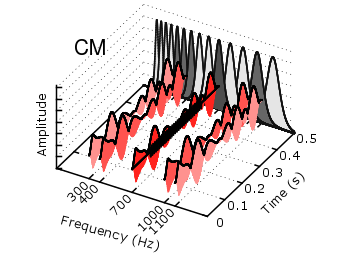Fig. 1 Visualization of the comodulation masking release using splot and special-filenames (code to produce this figure, gfb_loop.gnu, gfb.dat, noise.dat)

In Fig. 1 the end result is seen. What we have done is to replace the last `splot` command from the cmr.gnu file with the following code.

```set samples 500
# Define the sinusoid signal to be plotted
sig(y) = y>0.1 && y<0.4 ? 0.45*sin(2*pi*100*y)+2 : 2
# The desired range is 0:0.5, but the samples were created for the
# x-axis, which has a range of 0:1400, therefore we calculate an
# factor to do the plot
fact = 1400/0.5
splot '+' u (700):(\$1/fact):(sig(\$1/fact)) w l ls 14
```

We define the function `sig(y)` which is a 100Hz sinusoid centered at 2 for values of y between 0.1 and 0.4 and constant 2 else. In order to place this two dimensional function in our 3D plot we use the `special-filenames` property from Gnuplot, in this case the `'+'` variant. This tells Gnuplot to use the xrange, apply a sampling of it and return it as first column for the plot command. But for our plot we need the y-axis and not the x-axis, because the x values should be constant at 700 and are therefore given by `(700)` at the `splot` command. The values of the first column, given by `\$1` are scaled by `fact` in order to match the two axis and are then directly used as y values and given to the `sig(y)` function for the z values.

October 27th, 2010 | 11 Comments

If we have more than one graph that should be displayed in a figure, the `multiplot` command is the one to use in Gnuplot. But as we will see this is not a trivial task.
Let us consider we have four different functions that should be presented in the same figure as shown in Fig. 1.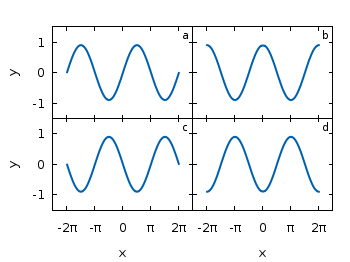Fig. 1 A multiplot with reduced axes labeling and nicely arranged graphs (code to produce this figure)

The functions are given by:

```# Functions (1/0 means not defined)
a = 0.9
f(x) = abs(x)<2*pi ? a*sin(x)           : 1/0
g(x) = abs(x)<2*pi ? a*sin(x+pi/2)      : 1/0
h(x) = abs(x)<2*pi ? a*sin(x+pi)        : 1/0
k(x) = abs(x)<2*pi ? a*sin(x+3.0/2*pi)  : 1/0
```

For an explanation of the used syntax to declare the functions have a look at the Defining piecewise functions article.

In a first attempt we just use the `multiplot` command:

```### Start multiplot (2x2 layout)
set multiplot layout 2,2 rowsfirst
# --- GRAPH a
set label 1 'a' at graph 0.92,0.9 font ',8'
plot f(x) with lines ls 1
# --- GRAPH b
set label 1 'b' at graph 0.92,0.9 font ',8'
plot g(x) with lines ls 1
# --- GRAPH c
set label 1 'c' at graph 0.92,0.9 font ',8'
plot h(x) with lines ls 1
# --- GRAPH d
set label 1 'd' at graph 0.92,0.9 font ',8'
plot k(x) with lines ls 1
unset multiplot
### End multiplot
```

We also added a label to every graph in order to identify them easily in the figure. Note that we overwrite the label 1 for every graph. If we don’t do that, then on the last graph all four labels will be present. Using this simple approach we will get Fig. 2. As you can see this is not an ideal case to use the space in the figure. The xtics and the ytics are just the same in every graph and are not needed to be displayed on every graph.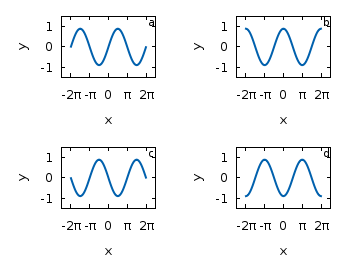Fig. 2 A straightforward use of the multiplot command to plot four different functions (code to produce this figure)

But before we fix this we will introduce the use of macros in order to shorten the code a lot. As you can see in the code block above, the set label command contains the same position for every graph. We can shorten this by:

```set macros
# Placement of the a,b,c,d labels in the graphs
POS = "at graph 0.92,0.9 font ',8'"
[...]
set label 1 'a' @POS
plot f(x) with lines ls 1
```

and so on for the other graphs.

But the macros are not only useful for the different labels, but also for the other settings of the multiplot.
First we want to remove the x/y-tics and labels, where they are not necessary. Here it is the same as with the label settings. Every graph uses the settings from the graph before, if we didn’t change these settings. In order to remove the xtics at a given graph we have to tell this explicitly. Therefore we define macros for the two cases label+tics vs. nolabel+notics:

```# x- and ytics for each row resp. column
NOXTICS = "set xtics ('' -2*pi,'' -pi,'' 0,'' pi,'' 2*pi); \
unset xlabel"
XTICS = "set xtics ('-2π' -2*pi,'-π' -pi,'0' 0,'π' pi,'2π' 2*pi);\
set xlabel 'x'"
NOYTICS = "set format y ''; unset ylabel"
YTICS = "set format y '%.0f'; set ylabel 'y'"
```

These will then be used in the plotting section:

```# --- GRAPH a
@NOXTICS; @YTICS
[...]
# --- GRAPH b
@NOXTICS; @NOYTICS
[...]
# --- GRAPH c
@XTICS; @YTICS
[...]
# --- GRAPH d
@XTICS; @NOYTICS
```

Applying the axes settings will result in Fig. 3.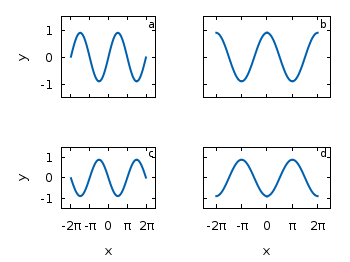Fig. 3 A multiplot with reduced axes labeling (code to produce this figure)

Now the label etc. on the axes are done in a nice way, but the sizes and the spaces between the individual graphs are very bad. This comes from the fact that Gnuplot calculates the size of a graph depending on the presence of tics and labels. In order to have graphs with the same size and align them without spaces between them we have to set the margins of the individual graphs manually.

```# Margins for each row resp. column
TMARGIN = "set tmargin at screen 0.90; set bmargin at screen 0.55"
BMARGIN = "set tmargin at screen 0.55; set bmargin at screen 0.20"
LMARGIN = "set lmargin at screen 0.15; set rmargin at screen 0.55"
RMARGIN = "set lmargin at screen 0.55; set rmargin at screen 0.95"
```

The trick is that we use the `at screen` command to arrange the margins absolutely in the figure. As you can see the bottom margin of the two figures in the top is placed at `0.55`, the same value the top margin of the two lower graphs start.
These margins are then added in the same way to the multiplot command as the label settings and we get:

```# --- GRAPH a
@TMARGIN; @LMARGIN
[...]
# --- GRAPH b
@TMARGIN; @RMARGIN
[...]
# --- GRAPH c
@BMARGIN; @LMARGIN
[...]
# --- GRAPH d
@BMARGIN; @RMARGIN
```

Having done all this we will finally get our desired figure which is shown in Fig. 1.

August 13th, 2010 | 7 Comments

In Gnuplot it is easy to define a continuous and differentiable function such as `f(x) = x`, but what to do if we need a function that fulfill non of these conditions?
For example let us consider a step function. Typically a step function is given by

```           / 1   if x > a
step(x) = -|
\ 0   else
```

In Gnuplot this can be achieved by using the ternary operator:

```step(x) = x>a ? 1 : 0
```

Which is a simple if-else statement and means `step(x)=1 if x>a else step(x)=0`.

If we plot this function we get Fig. 1.

Fig. 1 Continuous plot of the step function (code to produce this figure)

As you can see this will result in a continuous plot. If we want a discontinuity in the plot, we have to create two separate functions that are only piecewise defined. This can be achieved by using `1/0` that will result in a undefined value.

```f(x) = x<a  ? 1 : 1/0
g(x) = x>=a ? 1 : 1/0
```

Plotting both functions will result in Fig. 2.

Fig. 2 Discontinuous plot of the step function (code to produce this figure)

The ternary operator can also be used in an iterative way. For example if we want to define a rectangular function that is given by

```           / 0     if abs(x) > 0.5
rect(x) = -| 0.5   if abs(x) = 0.5
\ 1     if abs(x) < 0.5
```

we can use the following statement in Gnuplot to define it:

```rect(x) = abs(x)>0.5 ? 0 : abs(x)<0.5 ? 1 : 0.5
```

In Fig. 3 you can see a plot of this function. To produce the sharp edges of the rectangular function we use a higher number of sampling points (also in Fig. 1 for the step function). In order to plot a function Gnuplot calculates 100 points of the given function and draw a line through them. This can be set to another value with the `set samples <value>` command.

Fig. 3 Plot of the above defined rect(x) function (code to produce this figure)

April 27th, 2010 | 12 Comments

In this tutorial you will create your first gnuplot figure. Therefore open a text file in your preferred text editor, or directly download the code for this tutorial.
Firstly we have to tell gnuplot the output terminal we want to use. The most common are png, svg, postscript or LaTeX output, but first we will start with the output just on the screen using the wxWidgets toolkit. Therefore we have to write this line of code:

```set terminal wxt size 350,262 enhanced font 'Verdana,10' persist
```

After that we will specify the style of the output, because the default gnuplot output is ugly in many ways.

```# Line width of the axes
set border linewidth 1.5
# Line styles
set style line 1 linecolor rgb '#0060ad' linetype 1 linewidth 2
set style line 2 linecolor rgb '#dd181f' linetype 1 linewidth 2
```

You can see, a comment starts with a `#`. We use the `set` command and specify the border line width and two line styles with the number `1` and `2`. For them we specified a colour, the line type where 1 is a normal line and also the line width.

In our first graph we want to plot a sinusoid and a cosinus. Therefore we specify our functions and plot them:

```a = 0.9
f(x) = a * sin(x)
g(x) = a * cos(x)
# Plot
plot f(x) title 'sin(x)' with lines linestyle 1, \
g(x) notitle with lines linestyle 2
```

As you can see, the definitions of functions in gnuplot are straight forward. We want to plot more than one function that’s why we have to divide the two commands with a comma. The backslash tells gnuplot that we have a line break at this position.

Now we save our file as introduction.gnu and execute it by running the following command in BASH under Linux.

```\$ gnuplot introduction.gnu
```

Under Windows you can start gnuplot with the `wgnuplot.exe` file and then plot the introduction file by the following command.

```gnuplot> load 'path_to_your_file/introduction.gnu'
```

This should produce in both cases a graph as shown in Fig. 1.

Fig. 1 Our first ugly result (code to produce this figure)

As you can see, we have to tune it a little bit to get a nice graph. Therefore put the following code in front of the function’s definitions (in front of the plot command is sufficient, but I prefer to have the function’s definitions near the plot command).

```# Legend
set key at 6.1,1.3
# Axes label
set xlabel 'x'
set ylabel 'y'
# Axes ranges
set xrange [-2*pi:2*pi]
set yrange [-1.5:1.5]
# Axes tics
set xtics ('-2π' -2*pi, '-π' -pi, 0, 'π' pi, '2π' 2*pi)
set ytics 1
set tics scale 0.75
```

At first we set the legend to a specific position, put labels on the axes and set the ranges of the axes. After that, we specify the positions of the xtics and set strings (note that we can directly use utf-8 strings here) to the given positions. For the ytics we tell gnuplot to make a tic every multiple of 1. Also, we scale the length of the tics, because the default length is a bit too long. Now we can save the file, run the above command again and hopefully get the following figure.

Fig. 2 Our first very nice result (code to produce this figure)

As you have seen in this short tutorial, plotting a function with gnuplot is easy, you only have to tweak a little bit on the appearances of the figure.

Now you can have a look at how to plot data from a data file or how to use other outputs for the figures (png, svg, latex) than your screen.